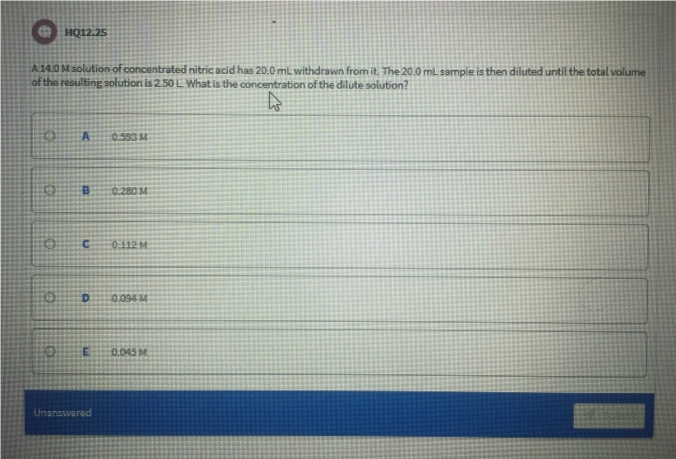# Problem: A 14.0 M solution of concentrated nitric acid has 20.0 mL withdrawn from it. The 20.0 mL sample is then diluted until the total volume of the resulting solution is 2.50L. What is the concentration of the dilute solution?a.  0.593 Mb. 0.280 Mc. 0.112 Md. 0.094 Me. 0.045 M

###### FREE Expert Solution
87% (446 ratings)###### Problem Details

A 14.0 M solution of concentrated nitric acid has 20.0 mL withdrawn from it. The 20.0 mL sample is then diluted until the total volume of the resulting solution is 2.50L. What is the concentration of the dilute solution?

a.  0.593 M

b. 0.280 M

c. 0.112 M

d. 0.094 M

e. 0.045 M